Calculus Volume 1

# 6.2Determining Volumes by Slicing

Calculus Volume 16.2 Determining Volumes by Slicing

### Learning Objectives

• 6.2.1 Determine the volume of a solid by integrating a cross-section (the slicing method).
• 6.2.2 Find the volume of a solid of revolution using the disk method.
• 6.2.3 Find the volume of a solid of revolution with a cavity using the washer method.

In the preceding section, we used definite integrals to find the area between two curves. In this section, we use definite integrals to find volumes of three-dimensional solids. We consider three approaches—slicing, disks, and washers—for finding these volumes, depending on the characteristics of the solid.

### Volume and the Slicing Method

Just as area is the numerical measure of a two-dimensional region, volume is the numerical measure of a three-dimensional solid. Most of us have computed volumes of solids by using basic geometric formulas. The volume of a rectangular solid, for example, can be computed by multiplying length, width, and height: $V=lwh.V=lwh.$ The formulas for the volume of a sphere $(V=43πr3),(V=43πr3),$ a cone $(V=13πr2h),(V=13πr2h),$ and a pyramid $(V=13Ah)(V=13Ah)$ have also been introduced. Although some of these formulas were derived using geometry alone, all these formulas can be obtained by using integration.

We can also calculate the volume of a cylinder. Although most of us think of a cylinder as having a circular base, such as a soup can or a metal rod, in mathematics the word cylinder has a more general meaning. To discuss cylinders in this more general context, we first need to define some vocabulary.

We define the cross-section of a solid to be the intersection of a plane with the solid. A cylinder is defined as any solid that can be generated by translating a plane region along a line perpendicular to the region, called the axis of the cylinder. Thus, all cross-sections perpendicular to the axis of a cylinder are identical. The solid shown in Figure 6.11 is an example of a cylinder with a noncircular base. To calculate the volume of a cylinder, then, we simply multiply the area of the cross-section by the height of the cylinder: $V=A·h.V=A·h.$ In the case of a right circular cylinder (soup can), this becomes $V=πr2h.V=πr2h.$

Figure 6.11 Each cross-section of a particular cylinder is identical to the others.

If a solid does not have a constant cross-section (and it is not one of the other basic solids), we may not have a formula for its volume. In this case, we can use a definite integral to calculate the volume of the solid. We do this by slicing the solid into pieces, estimating the volume of each slice, and then adding those estimated volumes together. The slices should all be parallel to one another, and when we put all the slices together, we should get the whole solid. Consider, for example, the solid S shown in Figure 6.12, extending along the $x-axis.x-axis.$

Figure 6.12 A solid with a varying cross-section.

We want to divide $SS$ into slices perpendicular to the $x-axis.x-axis.$ As we see later in the chapter, there may be times when we want to slice the solid in some other direction—say, with slices perpendicular to the y-axis. The decision of which way to slice the solid is very important. If we make the wrong choice, the computations can get quite messy. Later in the chapter, we examine some of these situations in detail and look at how to decide which way to slice the solid. For the purposes of this section, however, we use slices perpendicular to the $x-axis.x-axis.$

Because the cross-sectional area is not constant, we let $A(x)A(x)$ represent the area of the cross-section at point $x.x.$ Now let $P={x0,x1…,Xn}P={x0,x1…,Xn}$ be a regular partition of $[a,b],[a,b],$ and for $i=1,2,…n,i=1,2,…n,$ let $SiSi$ represent the slice of $SS$ stretching from $xi−1toxi.xi−1toxi.$ The following figure shows the sliced solid with $n=3.n=3.$

Figure 6.13 The solid $SS$ has been divided into three slices perpendicular to the $x-axis.x-axis.$

Finally, for $i=1,2,…n,i=1,2,…n,$ let $xi*xi*$ be an arbitrary point in $[xi−1,xi].[xi−1,xi].$ Then the volume of slice $SiSi$ can be estimated by $V(Si)≈A(xi*)Δx.V(Si)≈A(xi*)Δx.$ Adding these approximations together, we see the volume of the entire solid $SS$ can be approximated by

$V(S)≈∑i=1nA(xi*)Δx.V(S)≈∑i=1nA(xi*)Δx.$

By now, we can recognize this as a Riemann sum, and our next step is to take the limit as $n→∞.n→∞.$ Then we have

$V(S)=limn→∞∑i=1nA(xi*)Δx=∫abA(x)dx.V(S)=limn→∞∑i=1nA(xi*)Δx=∫abA(x)dx.$

The technique we have just described is called the slicing method. To apply it, we use the following strategy.

### Problem-Solving Strategy

#### Problem-Solving Strategy: Finding Volumes by the Slicing Method

1. Examine the solid and determine the shape of a cross-section of the solid. It is often helpful to draw a picture if one is not provided.
2. Determine a formula for the area of the cross-section.
3. Integrate the area formula over the appropriate interval to get the volume.

Recall that in this section, we assume the slices are perpendicular to the $x-axis.x-axis.$ Therefore, the area formula is in terms of x and the limits of integration lie on the $x-axis.x-axis.$ However, the problem-solving strategy shown here is valid regardless of how we choose to slice the solid.

### Example 6.6

#### Deriving the Formula for the Volume of a Pyramid

We know from geometry that the formula for the volume of a pyramid is $V=13Ah.V=13Ah.$ If the pyramid has a square base, this becomes $V=13a2h,V=13a2h,$ where $aa$ denotes the length of one side of the base. We are going to use the slicing method to derive this formula.

### Checkpoint6.6

Use the slicing method to derive the formula $V=13πr2hV=13πr2h$ for the volume of a circular cone.

### Solids of Revolution

If a region in a plane is revolved around a line in that plane, the resulting solid is called a solid of revolution, as shown in the following figure.

Figure 6.15 (a) This is the region that is revolved around the x-axis. (b) As the region begins to revolve around the axis, it sweeps out a solid of revolution. (c) This is the solid that results when the revolution is complete.

Solids of revolution are common in mechanical applications, such as machine parts produced by a lathe. We spend the rest of this section looking at solids of this type. The next example uses the slicing method to calculate the volume of a solid of revolution.

### Example 6.7

#### Using the Slicing Method to find the Volume of a Solid of Revolution

Use the slicing method to find the volume of the solid of revolution bounded by the graphs of $f(x)=x2−4x+5,x=1,andx=4,f(x)=x2−4x+5,x=1,andx=4,$ and rotated about the $x-axis.x-axis.$

### Checkpoint6.7

Use the method of slicing to find the volume of the solid of revolution formed by revolving the region between the graph of the function $f(x)=1/xf(x)=1/x$ and the $x-axisx-axis$ over the interval $[1,2][1,2]$ around the $x-axis.x-axis.$ See the following figure.### The Disk Method

When we use the slicing method with solids of revolution, it is often called the disk method because, for solids of revolution, the slices used to over approximate the volume of the solid are disks. To see this, consider the solid of revolution generated by revolving the region between the graph of the function $f(x)=(x−1)2+1f(x)=(x−1)2+1$ and the $x-axisx-axis$ over the interval $[−1,3][−1,3]$ around the $x-axis.x-axis.$ The graph of the function and a representative disk are shown in Figure 6.18(a) and (b). The region of revolution and the resulting solid are shown in Figure 6.18(c) and (d).

Figure 6.18 (a) A thin rectangle for approximating the area under a curve. (b) A representative disk formed by revolving the rectangle about the $x-axis.x-axis.$ (c) The region under the curve is revolved about the $x-axis,x-axis,$ resulting in (d) the solid of revolution.

We already used the formal Riemann sum development of the volume formula when we developed the slicing method. We know that

$V=∫abA(x)dx.V=∫abA(x)dx.$

The only difference with the disk method is that we know the formula for the cross-sectional area ahead of time; it is the area of a circle. This gives the following rule.

### Rule: The Disk Method

Let $f(x)f(x)$ be continuous and nonnegative. Define $RR$ as the region bounded above by the graph of $f(x),f(x),$ below by the $x-axis,x-axis,$ on the left by the line $x=a,x=a,$ and on the right by the line $x=b.x=b.$ Then, the volume of the solid of revolution formed by revolving $RR$ around the $x-axisx-axis$ is given by

$V=∫abπ[f(x)]2dx.V=∫abπ[f(x)]2dx.$
(6.3)

The volume of the solid we have been studying (Figure 6.18) is given by

$V=∫abπ[f(x)]2dx=∫−13π[(x−1)2+1]2dx=π∫−13[(x−1)4+2(x−1)2+1]dx=π[15(x−1)5+23(x−1)3+x]|−13=π[(325+163+3)−(−325−163−1)]=412π15units3.V=∫abπ[f(x)]2dx=∫−13π[(x−1)2+1]2dx=π∫−13[(x−1)4+2(x−1)2+1]dx=π[15(x−1)5+23(x−1)3+x]|−13=π[(325+163+3)−(−325−163−1)]=412π15units3.$

Let’s look at some examples.

### Example 6.8

#### Using the Disk Method to Find the Volume of a Solid of Revolution 1

Use the disk method to find the volume of the solid of revolution generated by rotating the region between the graph of $f(x)=xf(x)=x$ and the $x-axisx-axis$ over the interval $[1,4][1,4]$ around the $x-axis.x-axis.$

### Checkpoint6.8

Use the disk method to find the volume of the solid of revolution generated by rotating the region between the graph of $f(x)=4−xf(x)=4−x$ and the $x-axisx-axis$ over the interval $[0,4][0,4]$ around the $x-axis.x-axis.$

So far, our examples have all concerned regions revolved around the $x-axis,x-axis,$ but we can generate a solid of revolution by revolving a plane region around any horizontal or vertical line. In the next example, we look at a solid of revolution that has been generated by revolving a region around the $y-axis.y-axis.$ The mechanics of the disk method are nearly the same as when the $x-axisx-axis$ is the axis of revolution, but we express the function in terms of $yy$ and we integrate with respect to y as well. This is summarized in the following rule.

### Rule: The Disk Method for Solids of Revolution around the y-axis

Let $g(y)g(y)$ be continuous and nonnegative. Define $QQ$ as the region bounded on the right by the graph of $g(y),g(y),$ on the left by the $y-axis,y-axis,$ below by the line $y=c,y=c,$ and above by the line $y=d.y=d.$ Then, the volume of the solid of revolution formed by revolving $QQ$ around the $y-axisy-axis$ is given by

$V=∫cdπ[g(y)]2dy.V=∫cdπ[g(y)]2dy.$
(6.4)

The next example shows how this rule works in practice.

### Example 6.9

#### Using the Disk Method to Find the Volume of a Solid of Revolution 2

Let $RR$ be the region bounded by the graph of $g(y)=4−yg(y)=4−y$ and the $y-axisy-axis$ over the $y-axisy-axis$ interval $[0,4].[0,4].$ Use the disk method to find the volume of the solid of revolution generated by rotating $RR$ around the $y-axis.y-axis.$

### Checkpoint6.9

Use the disk method to find the volume of the solid of revolution generated by rotating the region between the graph of $g(y)=yg(y)=y$ and the $y-axisy-axis$ over the interval $[1,4][1,4]$ around the $y-axis.y-axis.$

### The Washer Method

Some solids of revolution have cavities in the middle; they are not solid all the way to the axis of revolution. Sometimes, this is just a result of the way the region of revolution is shaped with respect to the axis of revolution. In other cases, cavities arise when the region of revolution is defined as the region between the graphs of two functions. A third way this can happen is when an axis of revolution other than the $x-axisx-axis$ or $y-axisy-axis$ is selected.

When the solid of revolution has a cavity in the middle, the slices used to approximate the volume are not disks, but washers (disks with holes in the center). For example, consider the region bounded above by the graph of the function $f(x)=xf(x)=x$ and below by the graph of the function $g(x)=1g(x)=1$ over the interval $[1,4].[1,4].$ When this region is revolved around the $x-axis,x-axis,$ the result is a solid with a cavity in the middle, and the slices are washers. The graph of the function and a representative washer are shown in Figure 6.22(a) and (b). The region of revolution and the resulting solid are shown in Figure 6.22(c) and (d).

Figure 6.22 (a) A thin rectangle in the region between two curves. (b) A representative disk formed by revolving the rectangle about the $x-axis.x-axis.$ (c) The region between the curves over the given interval. (d) The resulting solid of revolution.

The cross-sectional area, then, is the area of the outer circle less the area of the inner circle. In this case,

$A(x)=π(x)2−π(1)2=π(x−1).A(x)=π(x)2−π(1)2=π(x−1).$

Then the volume of the solid is

$V=∫abA(x)dx=∫14π(x−1)dx=π[x22−x]|14=92πunits3.V=∫abA(x)dx=∫14π(x−1)dx=π[x22−x]|14=92πunits3.$

Generalizing this process gives the washer method.

### Rule: The Washer Method

Suppose $f(x)f(x)$ and $g(x)g(x)$ are continuous, nonnegative functions such that $f(x)≥g(x)f(x)≥g(x)$ over $[a,b].[a,b].$ Let $RR$ denote the region bounded above by the graph of $f(x),f(x),$ below by the graph of $g(x),g(x),$ on the left by the line $x=a,x=a,$ and on the right by the line $x=b.x=b.$ Then, the volume of the solid of revolution formed by revolving $RR$ around the $x-axisx-axis$ is given by

$V=∫abπ[(f(x))2−(g(x))2]dx.V=∫abπ[(f(x))2−(g(x))2]dx.$
(6.5)

### Example 6.10

#### Using the Washer Method

Find the volume of a solid of revolution formed by revolving the region bounded above by the graph of $f(x)=xf(x)=x$ and below by the graph of $g(x)=1/xg(x)=1/x$ over the interval $[1,4][1,4]$ around the $x-axis.x-axis.$

### Checkpoint6.10

Find the volume of a solid of revolution formed by revolving the region bounded by the graphs of $f(x)=xf(x)=x$ and $g(x)=1/xg(x)=1/x$ over the interval $[1,3][1,3]$ around the $x-axis.x-axis.$

As with the disk method, we can also apply the washer method to solids of revolution that result from revolving a region around the y-axis. In this case, the following rule applies.

### Rule: The Washer Method for Solids of Revolution around the y-axis

Suppose $u(y)u(y)$ and $v(y)v(y)$ are continuous, nonnegative functions such that $v(y)≤u(y)v(y)≤u(y)$ for $y∈[c,d].y∈[c,d].$ Let $QQ$ denote the region bounded on the right by the graph of $u(y),u(y),$ on the left by the graph of $v(y),v(y),$ below by the line $y=c,y=c,$ and above by the line $y=d.y=d.$ Then, the volume of the solid of revolution formed by revolving $QQ$ around the $y-axisy-axis$ is given by

$V=∫cdπ[(u(y))2−(v(y))2]dy.V=∫cdπ[(u(y))2−(v(y))2]dy.$

Rather than looking at an example of the washer method with the $y-axisy-axis$ as the axis of revolution, we now consider an example in which the axis of revolution is a line other than one of the two coordinate axes. The same general method applies, but you may have to visualize just how to describe the cross-sectional area of the volume.

### Example 6.11

#### The Washer Method with a Different Axis of Revolution

Find the volume of a solid of revolution formed by revolving the region bounded above by $f(x)=4−xf(x)=4−x$ and below by the $x-axisx-axis$ over the interval $[0,4][0,4]$ around the line $y=−2.y=−2.$

### Checkpoint6.11

Find the volume of a solid of revolution formed by revolving the region bounded above by the graph of $f(x)=x+2f(x)=x+2$ and below by the $x-axisx-axis$ over the interval $[0,3][0,3]$ around the line $y=−1.y=−1.$

### Section 6.2 Exercises

58.

Derive the formula for the volume of a sphere using the slicing method.

59.

Use the slicing method to derive the formula for the volume of a cone.

60.

Use the slicing method to derive the formula for the volume of a tetrahedron with side length $a.a.$

61.

Use the disk method to derive the formula for the volume of a trapezoidal cylinder.

62.

Explain when you would use the disk method versus the washer method. When are they interchangeable?

For the following exercises, draw a typical slice and find the volume using the slicing method for the given volume.

63.

A pyramid with height 6 units and square base of side 2 units, as pictured here.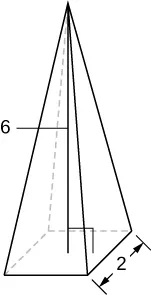64.

A pyramid with height 4 units and a rectangular base with length 2 units and width 3 units, as pictured here.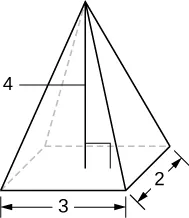65.

A tetrahedron with a base side of 4 units, as seen here.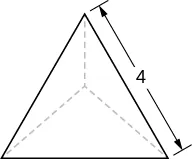66.

A pyramid with height 5 units, and an isosceles triangular base with lengths of 6 units and 8 units, as seen here.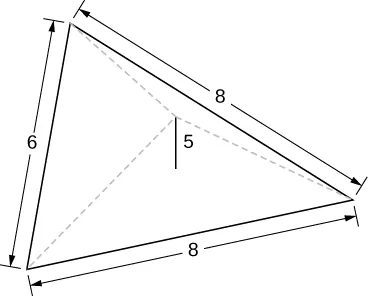67.

A cone of radius $rr$ and height $hh$ has a smaller cone of radius $r/2r/2$ and height $h/2h/2$ removed from the top, as seen here. The resulting solid is called a frustum.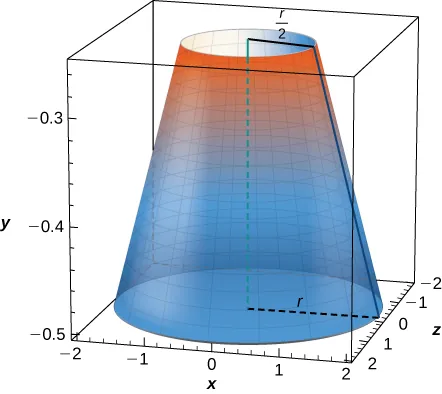For the following exercises, draw an outline of the solid and find the volume using the slicing method.

68.

The base is a circle of radius $a.a.$ The slices perpendicular to the base are squares.

69.

The base is a triangle with vertices $(0,0),(1,0),(0,0),(1,0),$ and $(0,1).(0,1).$ Slices perpendicular to the x-axis are semicircles.

70.

The base is the region under the parabola $y=1−x2y=1−x2$ in the first quadrant. Slices perpendicular to the xy-plane and parallel to the y-axis are squares.

71.

The base is the region under the parabola $y=1−x2y=1−x2$ and above the $x-axis.x-axis.$ Slices perpendicular to the $y-axisy-axis$ are squares.

72.

The base is the region enclosed by $y=x2y=x2$ and $y=9.y=9.$ Slices perpendicular to the x-axis are right isosceles triangles. The intersection of one of these slices and the base is the leg of the triangle.

73.

The base is the area between $y=xy=x$ and $y=x2.y=x2.$ Slices perpendicular to the x-axis are semicircles.

For the following exercises, draw the region bounded by the curves. Then, use the disk method to find the volume when the region is rotated around the x-axis.

74.

$x + y = 8 , x = 0 , and y = 0 x + y = 8 , x = 0 , and y = 0$

75.

$y = 2 x 2 , x = 0 , x = 4 , and y = 0 y = 2 x 2 , x = 0 , x = 4 , and y = 0$

76.

$y = e x + 1 , x = 0 , x = 1 , and y = 0 y = e x + 1 , x = 0 , x = 1 , and y = 0$

77.

$y = x 4 , x = 0 , and y = 1 for x ≥ 0 y = x 4 , x = 0 , and y = 1 for x ≥ 0$

78.

$y = x , x = 0 , x = 4 , and y = 0 y = x , x = 0 , x = 4 , and y = 0$

79.

$y = sin x , y = cos x , and x = 0 y = sin x , y = cos x , and x = 0$

80.

$y = 1 x , x = 2 , and y = 3 y = 1 x , x = 2 , and y = 3$

81.

$x 2 − y 2 = 9 and x + y = 9 , y = 0 and x = 0 x 2 − y 2 = 9 and x + y = 9 , y = 0 and x = 0$

For the following exercises, draw the region bounded by the curves. Then, find the volume when the region is rotated around the y-axis.

82.

$y = 4 − 1 2 x , x = 0 , and y = 0 y = 4 − 1 2 x , x = 0 , and y = 0$

83.

$y = 2 x 3 , x = 0 , x = 1 , and y = 0 y = 2 x 3 , x = 0 , x = 1 , and y = 0$

84.

$y = 3 x 2 , x = 0 , and y = 3 y = 3 x 2 , x = 0 , and y = 3$

85.

$y = 4 − x 2 , y = 0 , and x = 0 y = 4 − x 2 , y = 0 , and x = 0$

86.

$y = 1 x + 1 , x = 0 , x = 3 , and y = 0 y = 1 x + 1 , x = 0 , x = 3 , and y = 0$

87.

$x = sec ( y ) and y = π 4 , y = 0 and x = 0 x = sec ( y ) and y = π 4 , y = 0 and x = 0$

88.

$y = 1 x + 1 , x = 0 , , x = 2 , and y = 0 y = 1 x + 1 , x = 0 , , x = 2 , and y = 0$

89.

$y = 4 − x , y = x , and x = 0 y = 4 − x , y = x , and x = 0$

For the following exercises, draw the region bounded by the curves. Then, find the volume when the region is rotated around the x-axis.

90.

$y = x + 2 , y = x + 6 , x = 0 , and x = 5 y = x + 2 , y = x + 6 , x = 0 , and x = 5$

91.

$y = x 2 and y = x + 2 y = x 2 and y = x + 2$

92.

$x 2 = y 3 and x 3 = y 2 x 2 = y 3 and x 3 = y 2$

93.

$y = 4 − x 2 and y = 2 − x y = 4 − x 2 and y = 2 − x$

94.

[T] $y=cosx,y=e−x,x=0,andx=1.2927y=cosx,y=e−x,x=0,andx=1.2927$

95.

$y = x and y = x 2 y = x and y = x 2$

96.

$y = sin x , y = 5 sin x , x = 0 and x = π y = sin x , y = 5 sin x , x = 0 and x = π$

97.

$y = 1 + x 2 and y = 4 − x 2 y = 1 + x 2 and y = 4 − x 2$

For the following exercises, draw the region bounded by the curves. Then, use the washer method to find the volume when the region is revolved around the y-axis.

98.

$y = x , x = 4 , and y = 0 y = x , x = 4 , and y = 0$

99.

$y = x + 2 , y = 2 x − 1 , and x = 0 y = x + 2 , y = 2 x − 1 , and x = 0$

100.

$y = x 3 and y = x 3 y = x 3 and y = x 3$

101.

$x = e 2 y , x = y 2 , y = 0 , and y = ln ( 2 ) x = e 2 y , x = y 2 , y = 0 , and y = ln ( 2 )$

102.

$x = 9 − y 2 , x = e − y , y = 0 , and y = 3 x = 9 − y 2 , x = e − y , y = 0 , and y = 3$

103.

Yogurt containers can be shaped like frustums. Rotate the line $y=1mxy=1mx$ around the y-axis to find the volume between $y=aandy=b.y=aandy=b.$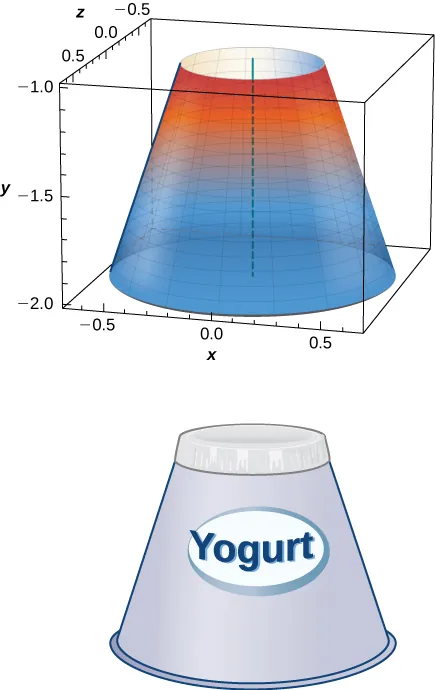104.

Rotate the ellipse $(x2/a2)+(y2/b2)=1(x2/a2)+(y2/b2)=1$ around the x-axis to approximate the volume of a football, as seen here.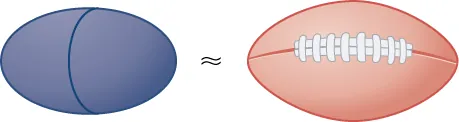105.

Rotate the ellipse $(x2/a2)+(y2/b2)=1(x2/a2)+(y2/b2)=1$ around the y-axis to approximate the volume of a football.

106.

A better approximation of the volume of a football is given by the solid that comes from rotating $y=sinxy=sinx$ around the x-axis from $x=0x=0$ to $x=π.x=π.$ What is the volume of this football approximation, as seen here?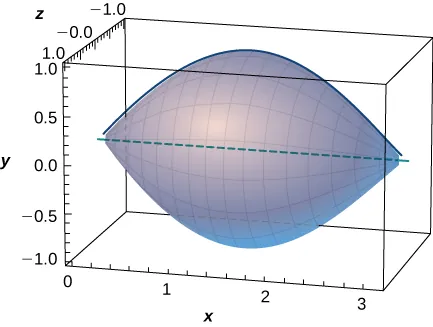107.

What is the volume of the Bundt cake that comes from rotating $y=sinxy=sinx$ around the y-axis from $x=0x=0$ to $x=π?x=π?$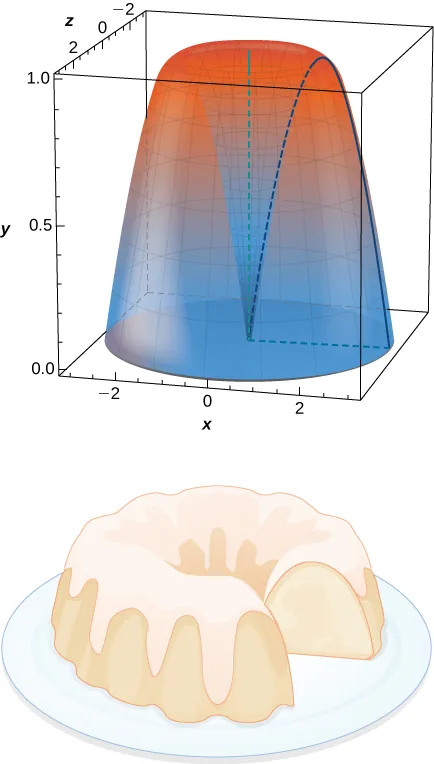For the following exercises, find the volume of the solid described.

108.

The base is the region between $y=xy=x$ and $y=x2.y=x2.$ Slices perpendicular to the x-axis are semicircles.

109.

The base is the region enclosed by the generic ellipse $(x2/a2)+(y2/b2)=1.(x2/a2)+(y2/b2)=1.$ Slices perpendicular to the x-axis are semicircles.

110.

Bore a hole of radius $aa$ down the axis of a right cone of height $bb$ and radius $bb$ through the base of the cone as seen here.111.

Find the volume common to two spheres of radius $rr$ with centers that are $2h2h$ apart, as shown here.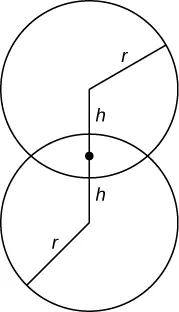112.

Find the volume of a spherical cap of height $hh$ and radius $rr$ where $h as seen here.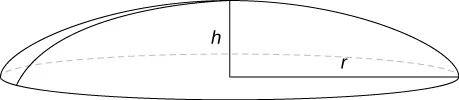113.

Find the volume of a sphere of radius $RR$ with a cap of height $hh$ removed from the top, as seen here.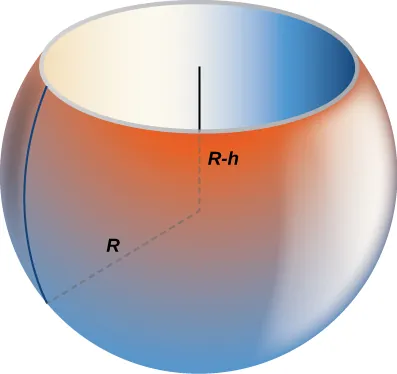Order a print copy

As an Amazon Associate we earn from qualifying purchases.